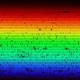# [易错题汇编]（十一）圆锥曲线篇

（1）已知$M(-2,0),N(2,0)$，动点$P$满足$|PM|+|PN|=4$，则动点$P$的轨迹是_______，动点$Q$满足$\big||QM|-|QN|\big|=4$，则动点$Q$的轨迹是_______；

（2）平面上的动点$P$到定点$F(1,0)$的距离比$P$到$y$轴的距离大$1$，那么动点$P$的轨迹是__________；

（3）与圆$x^2+y^2-4x=0$外切，且与$y$轴相切的动圆圆心的轨迹为__________；

（4）半径不等的两定圆$O_1,O_2$无公共点，动点$O$与圆$O_1,O_2$都内切，则圆心$O$的轨迹是__________．

（2）抛物线与一条射线；

（3）抛物线与一条去掉端点的射线；

（4）双曲线的一支或椭圆．

（1）抛物线$y=4x^2$的准线方程为_______；

（2）若$m$是$2$和$8$的等比中项，则圆锥曲线$x^2+\dfrac {y^2}{m}=1$的离心率为_______；

（3）若椭圆$\dfrac {x^2}{m}+y^2=1$的离心率为$\dfrac {\sqrt 3}{2}$，则$m$的值为_______；

（4）若双曲线$\dfrac {x^2}{a^2}-\dfrac {y^2}{b^2}=-1$的离心率为$\dfrac 54$，则它的两条渐近线的方程为__________；

（5）双曲线$\dfrac{x^2}{4}+\dfrac {y^2}{k}=1$的离心率为$e$，且$e\in(1,2)$，则$k$的取值范围是_______；

（6）双曲线$\dfrac {x^2}{16}-\dfrac {y^2}{9}=1$上的点$P$到点$(5,0)$的距离为$8.5$，则点$P$到$(-5,0)$的距离为_______．

（2）$\dfrac {\sqrt 3}{2}$或$\sqrt 5$；

（3）$4$或$\dfrac 14$；

（4）$\dfrac x3\pm\dfrac y4=0$；

（5）$(-12,0)$；

（6）$16.5$．

（1）过点$(0,1)$且与抛物线$y^2=2x$有且只有一个公共点的直线有____条;

（2）过点$(1,0)$且与双曲线$x^2-y^2=4$有且只有一个公共点的直线有___条；

（3）过双曲线$x^2-\dfrac {y^2}{2}=1$的右焦点作直线交双曲线于$A,B$两点，且$|AB|=4$，则这样的直线有_____条；

（4）双曲线$\dfrac {x^2}{9}-\dfrac {y^2}{4}=1$中，被点$P(2,1)$平分的弦所在的直线有______条；

（5）直线$k:y=kx-2$交抛物线$y^2=8x$于$A,B$两点，且$AB$中点的横坐标为$2$，则满足条件的直线$l$有_____条；

### 《[易错题汇编]（十一）圆锥曲线篇》有1条回应

1.OriBeta说：

第一大题的第三小题轨迹不含原点吧，那还能叫射线么